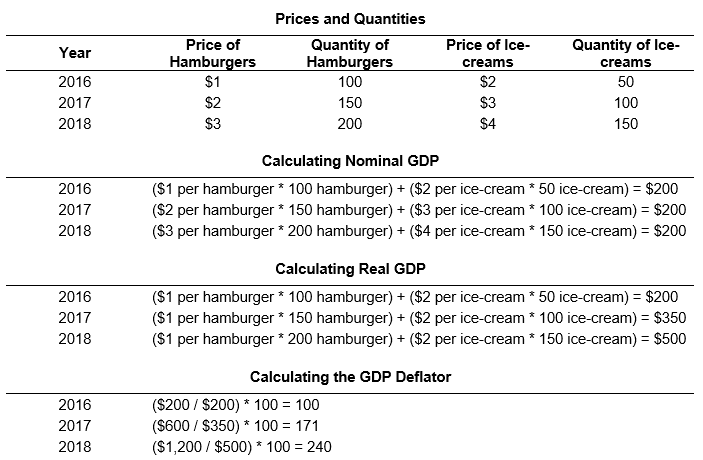# GDP Deflator Formula Calculator

Created by Tibor Pál, PhD candidate
Reviewed by Bogna Szyk and Adena Benn
Based on research by
Krugman, P.; Wells R. Economics, Fifth Edition (2017)
Last updated: Dec 10, 2022

This GDP deflator formula calculator measures the price level calculated as the ratio of nominal GDP to real GDP times 100. In other words, it helps you to determine the price level of all domestically produced final goods and services, also taking into account the exports of a country. Therefore, using the GDP deflator equation, you can calculate the inflation rate of an economy in the most comprehensive way.

## How to calculate GDP deflator?

The simple GDP deflation equation is the following:

GDP delfator = Nominal GDP / Real GDP × 100

To better understand the GDP deflator calculator, we need some understanding of what is real and nominal GDP. To do that, it might be a good idea to take a simplified numerical example.

Let's imagine a fictional economy called La-la-land, which produces only hamburgers and ice cream.

The table below shows the prices and quantities of the two goods produced in 2016, 2017, and 2018. To compute the nominal GDP of La-la-land, we need to multiply the amounts of hamburgers and ice cream by their prices in the given year.

However, if we would like to measure the amount produced that is not affected by price changes, we use constant prices for the calculation. This computation gives the real GDP, which is calculated each year by the base year's price, in our example, prices in 2016.

We use our GDP deflator formula to calculate the GDP deflator for each year. The resulting value shows how the price level of the produced hamburgers and ice creams has changed compared to the 2016 base year.With our results, we can find out the inflation rate associated with all produced goods in La-la-land. To do that, we need to utilize the inflation rate formula with the GDP deflators in different years.

Inflation rate in year 2 = (GDP deflator in year 2 – GDP deflator in year 1) / GDP deflator in year 1 × 100.

Substituting our numbers into the formula, the GDP deflator rose in 2017 from 100 to 171; the inflation rate is 100 × (171 – 100)/100, or 71 percent. In 2018, the GDP deflator rose to 240 from 171 the previous year, so the inflation rate is 100 × (240 – 171)/171, or 40 percent.

## Importance in economics

Since economies in real life are much more complicated than our oversimplified imaginary La-la-land, its good to remember that the GDP deflator gives you a picture of the changes in the price level in all factors of the Gross Domestic Product, namely investment and government expenditures, as well as for consumer spending and net exports.

Unlike other price indices, for example, the Consumer Price Index (CPI), the GDP deflator formula is not based on a fixed basket of goods and services. The basket is allowed to alter depending on the final consumption and investment in a given year. Therefore, it also reflects consumption and investment patterns. Specifically, for the GDP deflator, the basket in each year is the set of all goods that were produced domestically, weighted by the market value of the total consumption of each good. Therefore, new expenditure patterns are allowed to show up in the deflator as people respond to changing prices.

The theory behind this approach is that the GDP deflator reflects up-to-date expenditure patterns. For instance, if the price of pork increases relative to the price of beef, people may spend more money on pork as a substitute for beef.

In practice, the difference between the deflator and a price index like the Consumer Price Index (CPI) is often relatively small. On the other hand, with governments in developed countries increasingly utilizing price indexes for everything from fiscal and monetary planning to payments to social program recipients, even small differences between inflation measures can shift budget revenues and expenses by millions or billions of dollars.

Tibor Pál, PhD candidate
Nominal GDP
$million Real GDP$
million
GDP deflator
People also viewed…

### Books vs e-Books